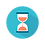From: Bayesian Models for Astrophysical Data, Cambridge Univ. Press

(c) 2017,  Joseph M. Hilbe, Rafael S. de Souza and Emille E. O. Ishida

you are kindly asked to include the complete citation if you used this material in a publication

Code 10.12 Bernoulli model in R using JAGS, for accessing the relationship between bulge size and the fraction of red spirals

===================================================================================

require(R2jags)

# Prepare data to JAGS
N <- nrow(Red)
x <- Red\$fracdeV
y <- Red\$type

# Construct data dictionary
X <- model.matrix(~ x,
data = Red)

K <- ncol(X)

logit_data <- list(Y = y,                          # response variable
X = X,                        # predictors
N = N,                        # sample size
K = K                         # number of columns
)

# Fit
LOGIT <-"model{
# Diffuse normal priors
for(i in 1:K){
beta[i] ~ dnorm(0, 1e-4)
}

# Likelihood function
for (i in 1:N){
Y[i] ~ dbern(p[i])
logit(p[i]) <- eta[i]
eta[i] <- inprod(beta[], X[i,])
}
}"

# Define initial values
inits <- function () {
list(beta = rnorm(ncol(X), 0, 0.1))
}

# Identify parameters
params <- c("beta"

# Fit
LOGIT_fit <- jags(data = logit_data,
inits = inits,
parameters = params,
model = textConnection(LOGIT),
n.thin = 1,
n.chains = 3,
n.burnin = 3000,
n.iter = 6000)

# Output
print(LOGIT_fit,intervals=c(0.025, 0.975),justify = "left", digits=2)

===================================================================================Output on screen:

Inference for Bugs model at "3", fit using jags,

3 chains, each with 6000 iterations (first 3000 discarded)

n.sims = 9000 iterations saved

mu.vect      sd.vect          2.5%      97.5%     Rhat        n.eff

beta             -4.87          0.23          -5.20        -4.55      1.02         140

beta              8.05          0.59           7.13          8.99      1.01         260

deviance     1911.16        44.72     1907.07     1915.70     1.00       9000

For each parameter, n.eff is a crude measure of effective sample size,

and Rhat is the potential scale reduction factor (at convergence, Rhat=1).

DIC info (using the rule, pD = var(deviance)/2)

pD = 1000.2 and DIC = 2911.4

DIC is an estimate of expected predictive error (lower deviance is better).